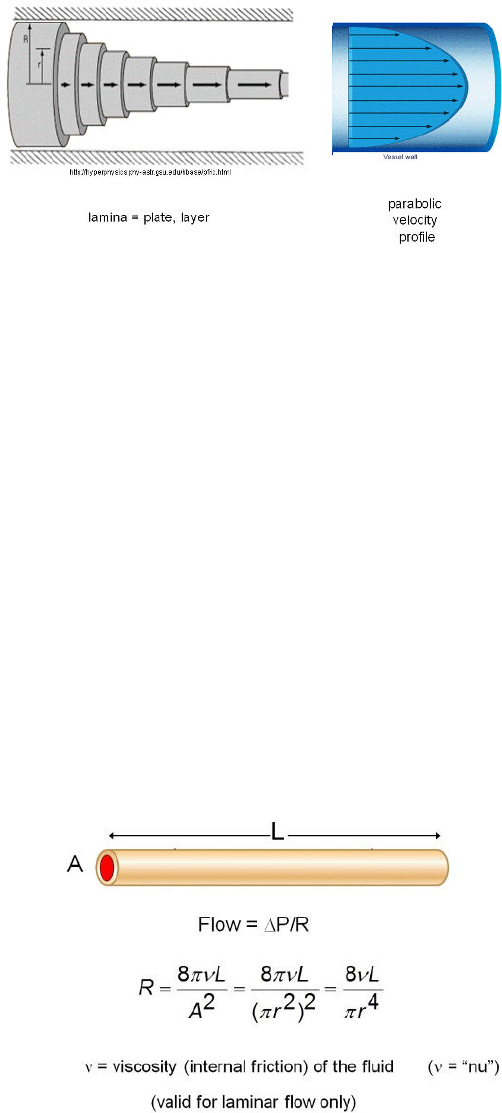Class Notes (1,100,000)
CA (630,000)
McGill (30,000)
PHGY (1,000)
PHGY 210 (400)
Lecture 17

# PHGY 210 Lecture Notes - Lecture 17: Endothelium, Endocardium, Pulmonary Vein

Department
Physiology
Course Code
PHGY 210
Professor
Melissa Vollrath
Lecture
17

This preview shows pages 1-2. to view the full 7 pages of the document.lecture #:
date:
professor:
17
February 10, 2017
Dr. Guevara
phgy 210

Only pages 1-2 are available for preview. Some parts have been intentionally blurred.PHGY 210 Lecture 17 Dr. Guevara
Do not redistribute. Page 2 of 7 Written by: Angelica Le
CARDIOVASCULAR
Last Lecture:
Flow through a vessel: If you increase the pressure at both ends, the perfusion pressure is unchanged and
flow is the same
Two things control flow: perfusion pressure and the vessel properties (viscosity, length, cross sectional area)
Can’t directly measure resistance, so find it using equation: Resistance = Perfusion pressure/flow
This Lecture:
LAMINAR OR PARABOLIC FLOW
Demonstrated using a collapsible umbrella holder
Each layer is called a lamina
The layers slide over each other, so the blood closest
to the midline moves the fastest and the fluid closest
to the walls moves the slowest.
There’s friction between the sliding layers, and this is
what gives the viscosity of the fluid
As fluid layers slide over each other, heat is being
generated and lost, and that energy comes from the
pressure energy
This is also why pressure falls as we move down the vessel, it’s giving up increasing amounts of energy in
heat to the fluid
Also called parabolic flow: if you look at the velocity as a function of the distance from the midline, you see
a parabola.
o At the walls of the vessel, the velocity is zero. As you move away from the walls, toward the midline of
the vessel, the velocity is increasing.
o Gives a shape of Y = X2, a parabola
It has a third name: Smooth Flow
o The fluid layers slide smoothly over each other
In many of the vessels in the body, this is the kind of flow you have
POISEUILLE’S LAW
Poiseuille wanted to figure out the resistance to flow, so he changed the perfusion pressure and realized:
Flow = Δ Perfusion Pressure P/Resistance to flow R
In a pipe or vessel, you can describe flow using cross sectional area and length of vessel
Demonstrates another way to find Resistance R (equation in the picture below)
A = cross sectional area, L = length, r = radius
Don’t memorize 8π, just know relationship between viscosity, length, cross sectional area, radius
If you blow through a pipe vs a straw of the same length,
using the same pressure, the flow in the straw is much slower
because of the cross sectional area
Aorta has a large cross sectional area to produce a smaller
resistance
The equation for flow here is only for laminar flow.
Turbulent flow is less smooth; equation doesn’t apply